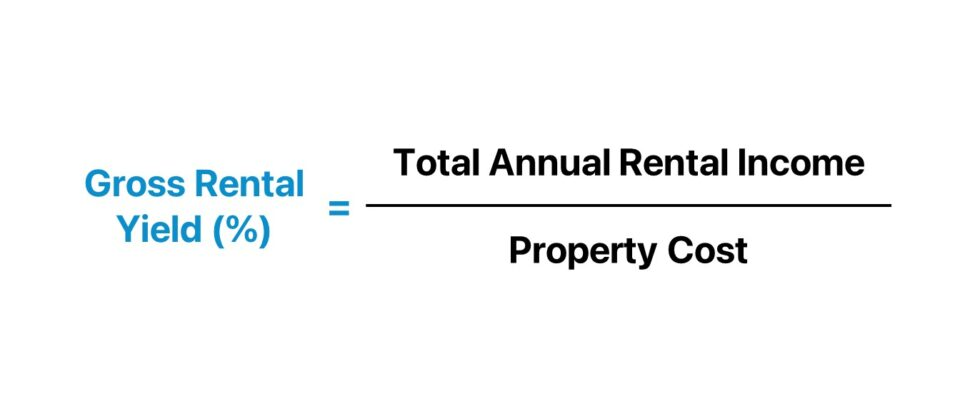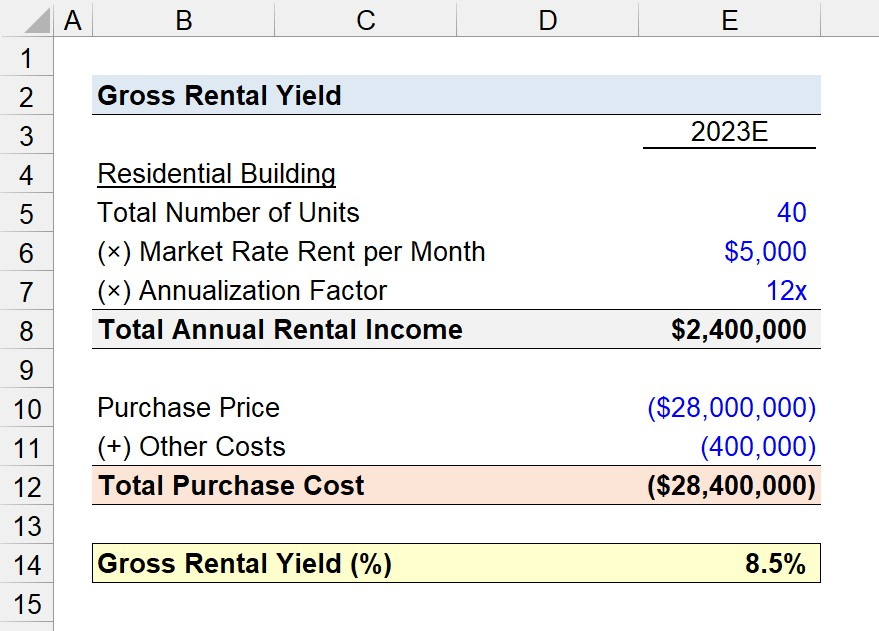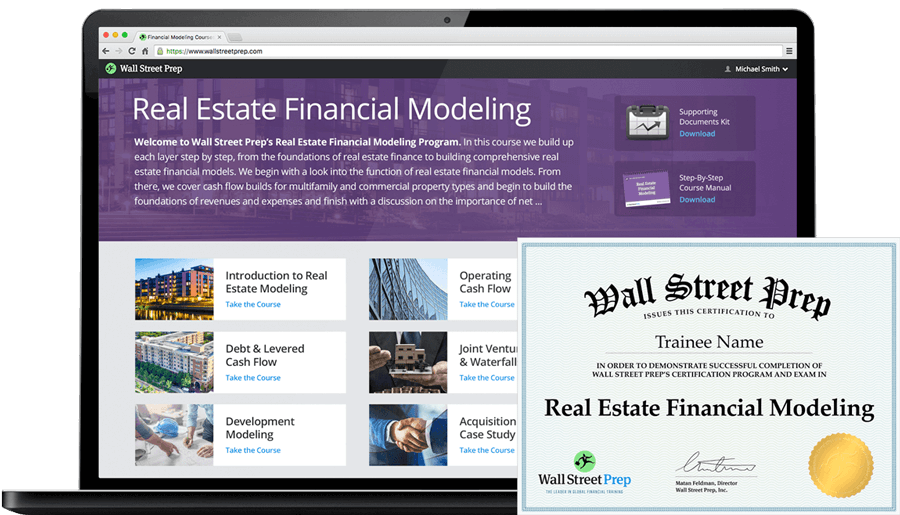Welcome to Wall Street Prep! Use code at checkout for 15% off.# Gross Rental Yield

Guide to Understanding Gross Rental Yield## How to Calculate Gross Rental Yield?

The gross rental yield, or “gross yield” for short, represents the return on a property investment prior to deducting operating expenses.

In general, the primary use-case of gross rental yield is to perform a “back of the napkin” analysis in order to determine if a property investment opportunity is worth spending more time on.

The gross rental yield is a quick method to confirm if the profit potential of a given property investment is enough.

If the potential return on a “gross” basis is insufficient to meet the minimum returns threshold specific to the real estate investor, then the probability of the return being appealing on a “net” basis is near zero.

In such scenarios, most rational investors would not proceed further in the investment evaluation process, since the property investment does not meet the returns criteria of the firm.

The calculation of the gross rental yield is straightforward, as the yield is simply the ratio between the total annual rental income and property cost.

1. Determine Total Annual Rental Income
2. Divide Total Annual Rental Income by the Property Cost
3. Convert into Percentage (Multiply by 100)

The higher the gross rental yield, the greater the potential income and return on the property investment (and vice versa).

However, the income component does not factor in operating expenses, so further analysis must be conducted before any decisions are made.## Gross Rental Yield vs. Net Rental Yield: What is the Difference?

In particular, there are two distinct types of rental yield metrics used by real estate investors and property owners.

• Gross Rental Yield – The gross rental yield, the simpler of the two metrics, estimates the potential return by comparing total rental income to the property cost. The notable drawback to gross rental yield is that the metric neglects expenses and operating costs.
• Net Rental Yield – In contrast, net rental yield – more commonly referred to as the capitalization rate, or “cap rate” – incorporates incurred operating costs.

The most common operating costs that the net rental yield factors into the return are the following:

• Property Management Fees
• Maintenance Costs (Repair, Renovation, etc.)
• Building Inspection Fees
• Property Taxes
• Property Insurance

Since the rental income component of the net yield is inclusive of operating costs, the implied return will be lower than the gross yield. Hence, the net rental yield is perceived as the more accurate view of the actual rental yield on a given property investment.

Another distinction between the two rental yield metrics lies in the denominator.

• Gross Rental Yield → “Total Property Cost”
• Net Rental Yield → “Property Value”

The denominator for the net rental yield is the appraised property value, so the metric reflects the return as of the present date.

Technically, the gross rental yield could also use the current property value, alike the net rental yield metric. The factor to consider is the timing and purpose of the analysis.

If an investor calculates the gross rental yield, it would be reasonable to assume that the investor is currently in the early stages of the potential acquisition, with limited access to historical operating data and no pro forma projections.

Given the context, the difference between the estimated purchase cost and current property value is unlikely to be significant. Therefore, the next step – assuming the gross yield appeals to the investor – is to delve deeper into conducting due diligence, which includes calculating the net rental yield among other return metrics.

## Gross Rental Yield Formula

The formula to calculate the gross rental yield is as follows.

Gross Rental Yield (%) = Total Annual Rental Income ÷ Property Cost
• Total Annual Rental Income → The total rental income expected to be generated by the property on an annualized basis.
• Property Cost → The sum of the purchase price of the property, including any origination fees and closing costs. Other expenses to include are those related to scheduled renovations or upgrades to the property post-acquisition.

To make comparisons among different properties easier, the resulting figure in decimal form should be multiplied by 100 to be converted into a percentage.

## Gross Rental Yield Calculator

We’ll now move on to a modeling exercise, which you can access by filling out the form below.#### Excel TemplateSubmitting...

## Gross Rental Yield Calculation Example

Suppose a real estate investor is offered the opportunity to acquire a residential building with a total of 40 units available for rent and a market rate rent of \$5,000 per month.

• Total Number of Units = 40 Units
• Market Rate Rent per Unit = \$5,000

The monthly rental income potential – assuming a vacancy rate of 0% – amounts to \$200,000, which we must annualize by multiplying by 12.

• Monthly Potential Rental Income = \$5,000 × 40 = \$200,000
• Annualization Factor = 12 Months
• Total Annual Rental Income = \$2,400,000

The total annual rental income, or potential gross income (PGI), comes out as \$2.4 million.

But to reiterate, the \$2.4 million income amount neglects operating costs, as well as vacancy and credit losses.

From there, we must determine the total purchase cost, which we’ll determine using the following assumptions.

• Property Purchase Price = \$28,000,000
• Other Costs = \$400,000

The sum of those two cost inputs amounts to a total purchase cost of \$28.4 million.

•   Total Purchase Cost = \$28,000,000 + \$400,000 = \$28,400,000

Upon dividing the total annual rental income by the total purchase cost, we arrive at a gross rental yield of 8.5%.

• Gross Rental Yield (%) = \$2.4 million ÷ \$28.4 million = 8.5%

By no means is the gross rental yield comprehensive enough on its own for an investor to truly grasp the risk-reward profile of a property investment. Thus, it is crucial to understand that the gross rental yield is a preliminary method to vet potential property investment opportunities, rather than guide investment decisions.20+ Hours of Online Video Training

### Master Real Estate Financial Modeling

This program breaks down everything you need to build and interpret real estate finance models. Used at the world's leading real estate private equity firms and academic institutions.

Inline Feedbacks